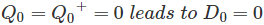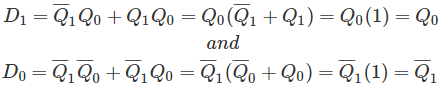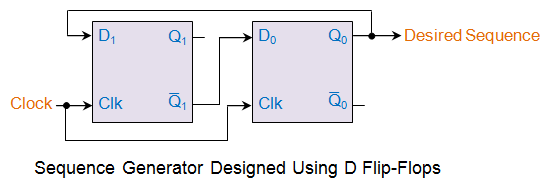# Sequence Generator

We all know that there are counters which pass through a definite number of states in a pre-determined order. For example, a 3-bit up-counter counts from 0 to 7 while the same order is reversed in the case of 3-bit down counter. These circuits when suitably manipulated can be made to count till an intermediate level also. This means that instead of counting till 7, we can terminate the process by resetting the counter just at, say, 5. Such counters are then known as mod-N counters. However, even this case, the order in which they count will not alter. But, what-if if we need to through a specific pattern which does not adhere to this standard way of counting? The solution would be to design a sequence generator.

This is because, the sequence generators are nothing but a set of digital circuits which are designed to result in a specific bit sequence at their output. There are several ways in which these circuits can be designed including those which are based on multiplexers and flip-flops. Here in this article we deal with the designing of sequence generator using D flip-flops (please note that even JK flip-flops can be made use of).
As an example, let us consider that we intend to design a circuit which moves through the states 0-1-3-2 before repeating the same pattern. The steps involved during this process are as follows.
Step 1
At first, we need to determine the number of flip-flops which would be required to achieve our objective. In our example, there are 4 states which are identical to the states of a 2-bit counter except the order in which they transit. From this, we can guess the requirement of flip-flops to be 2 in order to achieve our objective.

Step 2
Having this in mind, let us now write the state transition table for our sequence generator. This shown by the first four columns of Table I in which the first two columns indicate the present states while the next two columns indicate the corresponding next states. For instance, first state in our example is 0 = “00” which leads to the next state 1 = “01” (as shown by the gray shaded row in Table I).
Step 3
Now this state transition table is to be extended so as to include the excitation table of the flip-flop with which we desire to design our circuit. In our case, it is nothing but D flip-flop due to which we have the fifth and the sixth columns of the table representing the excitation table of D flip-flop.

For example, look at the orange shaded row in Table I in which the present and the next states 1 and 0 (respectively) result in D1 to be 0. The same row also shows the case whereinTable I

 Present States Next States Inputs of D flip-flops Q1 Q0 Q1+ Q0+ D1 D0 0 0 0 1 0 1 0 1 1 1 1 1 1 1 1 0 1 0 1 0 0 0 0 0

Step 4
Now its time to derive the Boolean expressions for D1 and D0. This can be done using any kind of simplification technique including K-map. However as our example is quite simple, we can just use the Boolean laws to solve for D1 and D0. ThusStep 5
Having known the inputs to either of the D flip-flops, now we can design our sequence generator as shown in this figure.In the circuit shown, the desired sequence is generated based on the clock pulses supplied. At this point, it should be noted that, the analogy presented here for a simple design can be effectively extended to generate longer sequence of bits.

Want To Learn Faster? 🎓
Get electrical articles delivered to your inbox every week.
No credit card required—it’s 100% free.